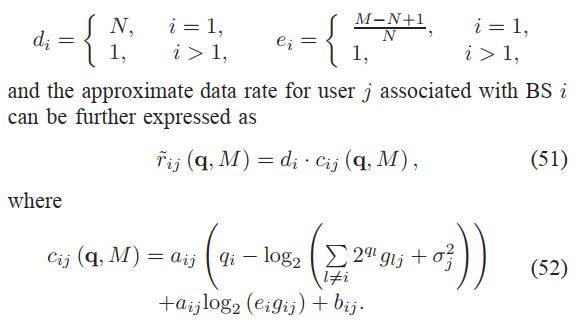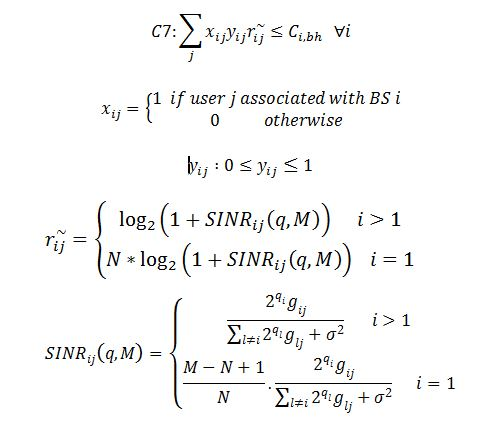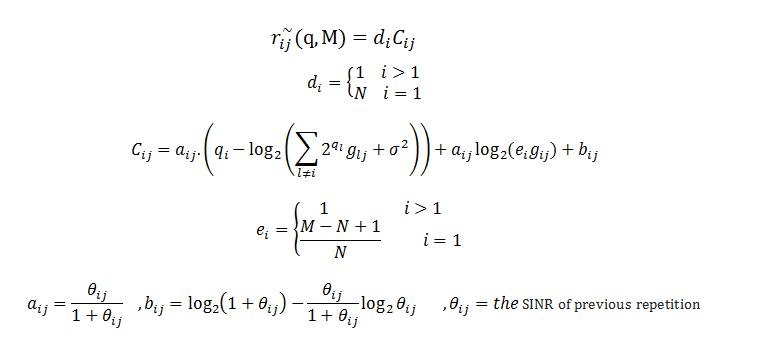Illegal operation: rel_entr( {positive constant}, {log-convex} )

(Rana) #1

Hello, I am trying to solve the following problem by cvx:
Cij=aij*(qi-log2(∑(l≠i)2^ql* gli))+ aij* log2((M+N-1)/N* gij)
M, q are the optimization variable that M is real variable and q(1,4).
By defining these variables the second part cij in cvx implementation is " cvx real affine expression (scalar)" .the type of M variable what should be that the second part of Cij be " cvx log-convex expression "?

(Mark L. Stone) #2

Can you please provide a complete reproducible example, complete with all code and input data?

Have you proven this is convex or concave?

help rel_entr

rel_entr Scalar relative entropy.
rel_entr(X,Y) returns an array of the same size as X+Y with the
relative entropy function applied to each element:
{ X.*LOG(X./Y) if X > 0 & Y > 0,
rel_entr(X,Y) = { 0 if X == 0 & Y >= 0,
{ +Inf otherwise.
X and Y must either be the same size, or one must be a scalar. If X and
Y are vectors, then SUM(rel_entr(X,Y)) returns their relative entropy.
If they are PDFs (that is, if X>=0, Y>=0, SUM(X)==1, SUM(Y)==1) then
this is equal to their Kullback-Liebler divergence SUM(KL_DIV(X,Y)).
-SUM(rel_entr(X,1)) returns the entropy of X.

Disciplined convex programming information:
rel_entr(X,Y) is convex in both X and Y, nonmonotonic in X, and
nonincreasing in Y. Thus when used in CVX expressions, X must be
real and affine and Y must be concave. The use of rel_entr(X,Y) in
an objective or constraint will effectively constrain both X and Y
constraints X >= 0 or Y >= 0 to enforce this.


So as you can see, the 2nd argument of rel_entr must be concave (or affine, which is a special case of concave), not log-convex as per your error message.

(Rana) #3

very thanks Mark,
the original function that i want simulate is:wherewhereand, 0<yij<1, xij={1 if user j is associated with BS i, 0 otherwise} , gij is positive number.
Due to the represents of the q variable in the numerator and the denominator of fraction , also the multiplication of the M variable is clear that it is not convex. In the appendix section mentioned,also:.
finally my cvx code is:
cvx_begin
variable q(1,Kt)
variable M
r_ij=zeros(Kt,Kr);
for e=1:Kr
for d=1:Kt
int=setdiff(Basic,d);
temp=2.^q(int)lossovernoise(int,e);
temp=temp+sigma2;
if d==1
di=N; ei=(M+N-1)/N;
else
di=1; ei=1;
end
Cij=a0_ij(d,e)
(q(d)-(-rel_entr(1,temp))/0.6931)+a0_ij(d,e)(-rel_entr(1,(eilossovernoise(d,e))))/0.6931+b0_ij(d,e);
r_ij(d,e)=di*Cij;
end
end
[Part_I,Part_II]=algorithm_one(PMax,UMin,UMax,Pa,epsilon,M,lambda,w,mu,r_ij,X_1,Y_1,Kr,Kt,q);
maximaize(Part_I-Part_II)
subject to
M<=Mmax
for c=1:Kt
end
for tt=1:Kt
sum(X_1(tt,:).*Y_1(tt,:).*r_ij(tt,:))<=Ci_BH(tt)
end
cvx_end

Error using cvx/rel_entr (line 71)
Disciplined convex programming error:
Illegal operation: rel_entr( {positive constant}, {log-convex} ).

Error in mainpart2017 (line 115)
Cij=a0_ij(d,e)(q(d)-(-rel_entr(1,temp))/0.6931)+a0_ij(d,e)(-rel_entr(1,(ei*lossovernoise(d,e))))/0.6931+b0_ij(d,e);

i have two another question ,too:
1: rel_entr can be used for any logarithmic function?
2:The ln function is implemented in cvx same as the logarithm, with this difference that it multiplied in 2.303 value?

(Mark L. Stone) #4

It appears that you have copied a problem out of the paper https://ieeexplore.ieee.org/stamp/stamp.jsp?arnumber=7990198 without understanding it.

Apparently, you are trying to solve the paper’s problem (33), which is proven to be a convex optimization problem in the paper’s Appendix B. The proof of convexity in that appendix provides a road map for how to formulate the problem in CVX, with use of log_sum_exp being the key.

You will need to read the paper more carefully in order to understand how the solution to problem (33) fits into a larger algorithm (algorithm 1) for solving a higher level non-convex problem of interest.

Your use of rel_entr is as a substitute for log, as described in CVXQUAD: How to use CVXQUAD's Pade Approximant instead of CVX's unreliable Successive Approximation for GP mode, log, exp, entr, rel_entr, kl_div, log_det, det_rootn, exponential cone. CVXQUAD's Quantum (Matrix) Entropy & Matrix Log related functions . In your case, I don’t recommend distracting yourself with that until you get a formulation which is accepted by CVX. Also niote that instances of log occurring in what will need to be handled via log_sum_exp, should indeed be handled by log_sum_exp, without use of rel_entr.

Also note that terms of the form 2^x will need to replaced by exp(log(2)*x) when determining the argument for log_sum_exp.

(Rana) #5

exactly, i want simulate this paper as a rival for my proposed method. i apply your recommendation about log_sum_exp.thank you so much for helping me.

(Rana) #6

Dear mark, the problem in the paper’s Appendix B is solved with log_sum_exp and output of the problem(51) was concave.Now my problem is that in problem(33) how to calculate the logarithm of a concave term while log_sum_exp and rel_enter can not be used?

(Mark L. Stone) #7

log_sum_exp is convex, so its negative is concave. The problem basically is log((-log_sum_exp), which is log of a concave argument, which is concave and is allowed by CVX. That’s why I wrote that Appendix B provides a road map for how to formulate the problem in CVX,

(Rana) #8

I sincerely appreciate your guidance and time. I couldn’t handle this problem yet. How are constraints 2 and 3 possible({concave} <= {real constant})? And that how 2^q is written in cvx, is a particular function used?

(Mark L. Stone) #9

What are constraints 2 and 3? Equations (2) and (3) in the paper are calculational formulas, not constraints.

q should be declared a variable in CVX, and then you should be able to enter 2^q. For use in log_sum_exp, you need to rewrite 2^q as exp(log(2)*q), which puts it into the form of a standard exponential, which provides the argument you need for log_sum_exp.

(Rana) #10

constraint of Equation(33) were meant.

(Mark L. Stone) #11

Constraint C6 can be entered " as is".

Constraint C7 can also be entered as is because it is affine because SINR is treated as input (numerical) data for this problem. In the outer algorithm (outside of an individual CVX invocation), SINR is adjusted based on the optimal values of q and M from the previous CVX invocation, and must be initialized before the first CVX invocation, as outlined in Algorithm 1 on the top right-hand side of p. 4725.

(Rana) #12

So the exp(log(2)*x) is not necessary used? In C6 if 2^q entered as is, the output of my code is convex for this case while mentioned in the appendix that the second term of f˜1 (q, M) is also a concave function .
In the case of C7, the SINR of previous repetition do not used and calculated with the equation (32).are you agree with me?

(Mark L. Stone) #13

exp(log(2)*x) is needed to get the argument of log_sum_exp, which is in the objective function.

For C7, I’ve now lost track of what the variable(s) are. What are x and y? If \tilde{r_{ij}} is calculated using initialized or updated SINR, then unless x or y are variables, what is? I will let you sort out the mess because I don’ have the energy to read the paper carefully enough.

(Rana) #14

X and Y calculated via algorithm 2. According to algorithm 4, First, Algorithms 2 and 3 run then the algorithm 1. the optimization variables are M and q vector .

(Mark L. Stone) #15

I leace you to sort through how the decision (optimization) variablesq and M come into play in constraint C7. You need to determine an explicit formulation for the formulation of problem (33) in CVX.

(Rana) #16In the Appendix, it is also written that:.